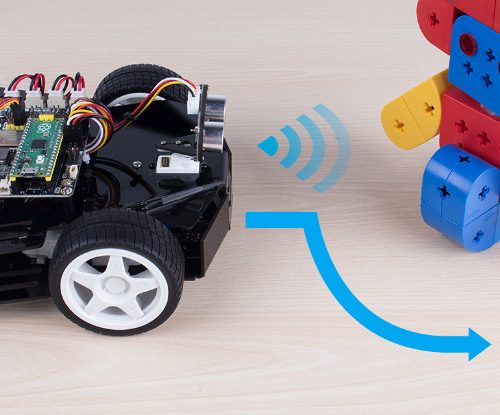# Obstacle Avoid¶Let Pico-4wd do a challenging task: automatic obstacle avoidance! Instead of simply stepping back when an obstacle is detected, the radar scans the surrounding area to find the widest road.

You can build a goal of the right size and watch it find the right place and slowly cross it.

## Code¶

Note

• Open the `obstacle_avoid.py` file under the path of `pico_4wd_car\examples` or copy this code into Thonny, then click “Run Current Script” or simply press F5 to run it.

• Don’t forget to click on the “MicroPython (Raspberry Pi Pico)” interpreter in the bottom right corner.

• For detailed tutorials, please refer to Open and Run Code Directly.

```import pico_4wd as car
import time

MOTOR_FORWARD_POWER = 30
MOTOR_TURNING_POWER = 50

FORWARD_SCAN_ANGLE = 50

status = "Danger"

# get scan status of 0, 1
# Split 0, leaves the free path
# print(paths)
# print("paths: %s" % paths)
length_list = []
# Calculate where is the widest
for path in paths:
length_list.append(len(path))
if max(length_list) < 4:
return "left"
# Calculate the direction of the widest
i = length_list.index(max(length_list))
pos += (len(paths[i]) - 1) / 2

if pos < delta:
return "right"
elif pos > 2 * delta:
return "left"
else:
return "forward"

def main():
# General
while True:
# If radar data return a int, means scan not finished, and the int is current angle status
if radar_data == 0 and status != "Danger":
print("Danger!")
status = "Danger"
car.move("stop")
continue
else:
status = "Save"

if direction == "left":
print("turn left")
time.sleep(0.5)
car.move("left", MOTOR_TURNING_POWER)
while True:
if status == 1:
break
car.move("stop")
elif direction == "right":
print("turn right")
time.sleep(0.5)
car.move("right", MOTOR_TURNING_POWER)
while True:
if status == 1:
break
car.move("stop")